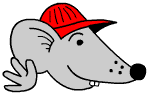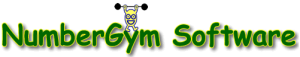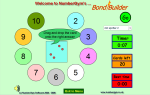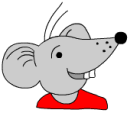## Free Y6 maths worksheets: Mass and weight

Mass and weightHow big is a tonne?
Can you picture a tonne of something? Not many people can do this but this worksheet tries to give some help. A tonne of water would fit exactly into a metre cube container!
What is the difference between mass and weight?
Continue reading “Free Y6 maths worksheets: Mass and weight”

## Free Y5 maths worksheet: Converting units

Free Y5 maths worksheet: Converting unitsJust a quick exercise in converting metric units from small to large and vice versa. This is not easy and as stated before a good understanding of both the metric system and place value is needed to be able to do this.
It is probably easier to convert large units to smaller:
Eg 3.5 litres = 3 500 ml
Continue reading “Free Y5 maths worksheet: Converting units”

## Free Y4 maths worksheet: Using metric units

Free Y4 maths worksheet: Using metric unitsScales are needed to carry out the activities on this worksheet which concentrates on using grams and kilograms. For measuring in grams ordinary kitchen scales are fine. Again, before measuring make an estimate. This is where cooking really comes into its own for maths activities. Measuring out ingredients for cakes, weighing pasta etc, measuring the amount of water to cook rice in – the list is endless.
More difficult is weighing in kilograms. Here a set of bathroom scales is better – but make sure they are using metric and not imperial measures. A good practical example is to weigh a suitcase before travelling abroad to make sure that it is under the baggage limit.

## News: Every Child Counts

Maths News: Every Child Counts

Every Child Counts

Secretary of State for Children, Ed Balls, has announced the roll out of a new programme of intensive support and one-to-one tuition in maths for primary children. The Government will invest £144 million over the next three years into rolling out nationally the Every Child A Reader (ECAR) and Every Child Counts (ECC) programmes. Continue reading “News: Every Child Counts”

## Free Y3 maths worksheets: Measuring in kilometres

Free Y3 maths worksheets: Measuring in kilometresThe first of these worksheets looks at metres and kilometres. Children will need to know that there are 1 000 metres in a kilometre.
Once this is known then the number of metres and half a kilometre and quarter of a kilometre can be worked out.
Half a km = 500m
Quarter of a km = 250m.
Continue reading “Free Y3 maths worksheets: Measuring in kilometres”

## Maths Game: Cross number 1

Maths Game: Cross number 1

Maths Game: Cross number 1

This is the first in a little series of fun maths games called cross numbers. Just like a crossword but with numbers. This first cross number is addition only, using mainly 2-digit numbers.

Drag the numbers from the left hand side into the correct squares on the cross number. You can check your answer by clicking on the Answer button.

## Free Y2 maths worksheets: Comparing and measuring lines

Free Y2 maths worksheets: Comparing and measuring linesThe ruler, with whole centimetres on, is introduced in Year 2. It is possible, but not easy, to get rulers with just cm on. You will probably have to make do with a ruler with both cm and mm on. These two worksheets look at short, straight lines and ask for a comparison: which is longest? Your children can measure these lines with a ruler but before they do so always ask them to have a guess (or estimate) about how long they think they are in centimetres. This is a habit which they should get into early and always keep!
When printing these free maths worksheets, make sure that you do not have the option ‘fit to page’ or similar page scaling options on, as this could affect the length of the lines when printing. All the free maths worksheets have been produced as A4 pages.

Free Y2 maths worksheet: Comparing and measuring lines (pg 2)

## Free Y1 maths worksheets: Comparing measurements

Free Y1 maths worksheets: Comparing measurementsThis week we are going to have another look at measurement and standard metric units of measurement. However, in year 1 measurement is much more about comparisons.
The first maths worksheet looks at vocabulary to do with comparing length:
Widest/narrowest, longest/shortest and tallest/shortest.
The second free maths worksheet looks at capacity where three terms are introduced: full, empty, fullest, emptiest and half full.
Plenty of practical work can be done at home with water, filling and emptying jugs and containers, whether it is in the sink, in the bath or outside using sand. (I’ve just been to St Ives, making sand castles – perfect opportunity!)
Make the most of this by talking with your children – get them to pour from one container to another – ask which has more; we are all often tricked into thinking that a tall narrow glass holds more than a short, wide glass etc.
Free Y1 maths worksheet: Comparisons of length

Free Y1 maths worksheet: Comparing capacity (pg 1)

## Number Gym: software review

Number Gym: software review19/30 Number Gym

Number Gym aims to improve children’s mental skills and understanding and has some unique features. There are two CDs evaluated here:
1.Bond Builder and Table TrainerBond Builder starts with sets of coloured dot patterns which can be counted or added. There is a circle of numbers around the outside, in the middle the set of dots. Drag the sum onto the correct answer on the outside circle of numbers. Timed with a best score facility.
Table Trainer is similar. Activities get harder as the tables get harder – eg on early games the set of numbers remain the same. On later games the values in the circles change. Also extension eg from knowing 7 x 6 = 42 to knowing 0.7 x 600. Continue reading “Number Gym: software review”

## Free Y6 maths worksheets: Revise doubling and halving

Free Y6 maths worksheets: Revise doubling and halvingOne of the most powerful tools children can have at their fingertips is the ability to double and halve numbers in their heads. These two maths worksheets look at doubling and halving 3-digit numbers.

A good approach to doubling a number such as 174 is:

1. double the hundred (200)

2. then double the 70 (140, making 340 in total)

3. then double the 4 (8, making 348 in total)

It seems that most people would tackle this by starting with the hundreds: the opposite of doing it on paper when you would start with the units. Continue reading “Free Y6 maths worksheets: Revise doubling and halving”